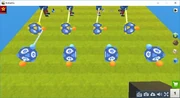A logic animation system is mostly made with the following logic cubes: Delay Cube, Cube Model Hider, Pressure Plate or Lever, and the models you want to animate.

## Animation of model

We'll first start by 4 frames of the animation, so 4 models. Let's explain the first image.

The number of frames of the animation can be as much as you want/as much as necessary. The Cube model hider's yellow logic connector can only be connected to models, follow the image's example. Each yellow logic connector from Cube model hider should be connected to a model.

Now connect the Delay cubes to Cube model hiders as the third image shows.First Delay Cube connected to first Cube Model Hider, second Delay Cube connected to second Cube Model Hider, etc.

And now the harder part. For orientation, we as first edit delay at model, which will begin in the animation (watch fourth image for understand). Also it means we set delay on 0. Of course, thats still example, you can add delay at start also.